# Privalov operators

Privalov parameters

Operators that allow one to express the condition of harmonicity of a function without using partial derivatives (cf. Harmonic function). Letbe a locally integrable function in a bounded domainof a Euclidean space,; let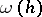denote the volume of the ballof radiuswith centre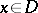, lying in; and letThe upper and lower Privalov operators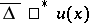and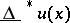are defined, respectively, by the formulas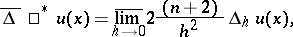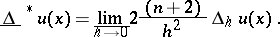If the upper and lower Privalov operators coincide, then the Privalov operatoris defined by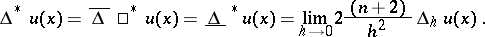If the functionhas continuous partial derivatives up to and including the second order at, then the Privalov operator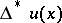exists atand is equal to the value of the Laplace operator: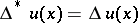. Privalov's theorem says: If a function, continuous in a domain, satisfies everywhere inthe conditions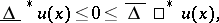thenis harmonic in. This implies that a function, continuous in, is harmonic if and only if at every pointone has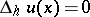, from some sufficiently smallonwards, or, in other words, if and only if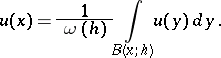The average value over the volume of a sphere can be replaced by that over the surface area.